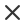SzűrőMédiatípus
Licenc típus
Orientáció
Stílus
Kizárás

# Matematikai stock-vektorok és illusztrációk

Seamless pattern on the theme of the subject of geometry formulas and charts of theorems a bright outline on a blue backgroundMath education vector pattern with handwritten formulas tasks plots calculations and geometrical figures vector illustrationSeamless pattern on the theme of the subject of geometry formulas and charts of theorems a bright outline on a dark backgroundMathematic vector seamless pattern with different signs figures and formulas back to school seamless scientific backgroundMath linear mathematics education circle background with geometrical plots formulas and calculations design concept vector illustrationPhysical vector seamless pattern with scientific formulas research equations and figures handwritten with chalk on a grey board seamless designMath formula mathematics calculus on school blackboard algebra and geometry science chalk pattern vector education concept scientific analysis number calculation complex knowledgeMath education vector pattern with handwritten formulas tasks plots calculations and geometrical figures vector illustrationMath theory mathematics calculus on class chalkboard algebra and geometry science handwritten formulas vector education concept formula and theory on blackboard science study illustrationPhysical formulas and phenomenon hand drawn illustration science board with math physics education at school vector illustrationMath linear mathematics education circle background with geometrical plots formulas and calculations design concept vector illustrationAbstract seamless pattern with black handwritten text mathematical formulas and equations and geometrical figures vector repeating background in style from the diary of a scientistCreative vector illustration of math equation mathematical arithmetic physics formulas background art design screen blackboard template abstract concept graphic elementYoung woman sitting on the floor and solving math problem with black board female person dressed in casual clothes study at home vector flat color illustrationMathematical vector scientific technical seamless pattern with handwritten formulas calculations equations on a green backgroundComplicate lab research derivatives manuscript complex test task plot isolated on white backdrop freehand linear black ink hand drawn draft in retro grungy scribble graph sketch style pencil on sheetMathematical vector seamless pattern with angle protractors triangles drawing rulers formulas and calculations on a green backgroundProgramming technical seamless pattern with programming code program flow diagrams formulas technical devices and schemes endless computer texture you can use any color of backgroundEndless mathematical texture with geometrical figures equations and calculations handwritten with chalk on a grey blackboard shuffled together mathematical vector seamless patternVector seamless geometry formula blackboard pattern made from hand drawn writings retro graphic style useful for scientific school and education designHand drawn math symbols icon in flat style mathematics formula vector illustration on isolated background school education sign business conceptPhysical formulas graphics and scientific calculations on chalkboard vintage hand drawn illustration laboratory seamless patternSchool seamless vector doodle pattern with different mathematical formulas and values good background for school printed supplies stationery other education stuffSeamless background on the topic of mathematical theorems symbols and formulas light contour on a green backgroundVector seamless formula pattern with black doodles made from hand drawn pen writings useful for scientific school and education designMathematical vector seamless pattern with figures formulas plots geometry tasks and other calculations handwritten on the copybook paper different colorsVector seamless pattern with handwritten white mathematics formulas figures calculations handwritings on the dark gray black squared paper geometry and algebra back to schoolEndless mathematical texture with geometrical figures plots and equations handwritten with chalk on a grey blackboard mathematical vector seamless patternMathematical vector seamless pattern with geometrical figures plots formulas other mathematical calculations you can use any color of background